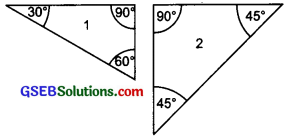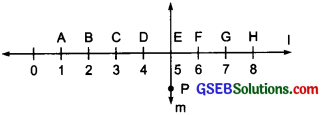# GSEB Solutions Class 6 Maths Chapter 5 Understanding Elementary Shapes Ex 5.5

Gujarat Board GSEB Textbook Solutions Class 6 Maths Chapter 5 Understanding Elementary Shapes Ex 5.5 Textbook Questions and Answers.

## Gujarat Board Textbook Solutions Class 6 Maths Chapter 5 Understanding Elementary Shapes Ex 5.5

Question 1.
Which of the following are models for perpendicular lines?
(a) The adjacent edges of a table top.
(b) The lines of a railway track.
(c) The line segments forming the letter ‘L’.
(d) The letter V.
Solution:
(a) Yes, the adjacent edges of a table top make a model of perpendicular lines.
(b) No, since parallel lines never intersect Railway track lines do not make a model of perpendicular lines.
(c) Yes, the line segments forming L are perpendicular lines.
(d) No, the line segments forming V, do not represent perpendicular lines.

Question 2.
Let $$\overline{\mathrm{OP}}$$ be the perpendicular to the line segment $$\overline{\mathrm{XY}}$$. Let $$\overline{\mathrm{PQ}}$$ and $$\overline{\mathrm{XY}}$$ intersect in the point A. What is the measure of $$\angle PAY$$?
Solution:
Since, $$\overline{\mathrm{OP}}$$ ⊥ $$\overline{\mathrm{XY}}$$.
Angle between them = 90°
Thus. $$\angle PAY$$ = 90°Question 3.
There are two set-squares in your box. What are the measures of the angles that are formed at their corners? Do they hive any angle measure that is common?
Solution:
The triangles formed by the corners of the two set-squares are given below:The measures of angles of triangle 1 are: 30°, 60° and 90°
The measures of angles of triangle 2 are: 45°, 45° and 90°
Yes, they have 90° as a common angle.

Question 4.
Study the diagram. The line l is perpendicular to line m(a) is CE = EG?
(b) Does PE bisect CG?
(c) Identify any two line segments for which PE is the perpendicular bisector.
(d) Ar these true?
(i) AC > FG
(ii) CD = GH
(iii) BC < EH
Solution:
(a) EC = 2 units, EG = 2 units
CE = EG
(b) Yes, PE bisects CG.
(c) $$\overline{\mathrm{BH}}$$ and $$\overline{\mathrm{DF}}$$
(d) (i) Yes
(ii) Yes
(iii) Yes